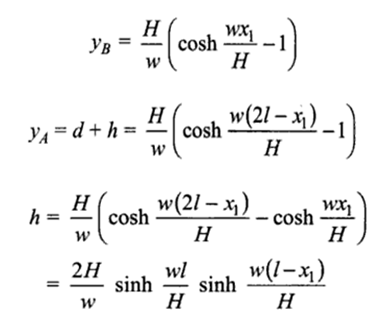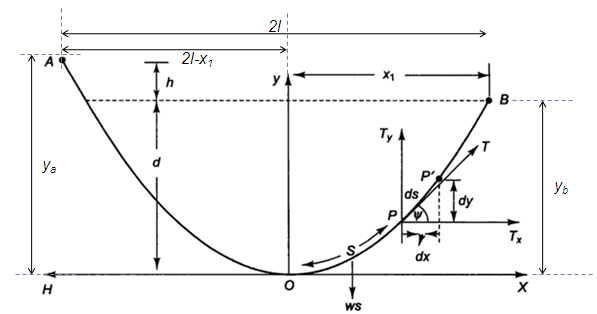# Sag of Transmission Line with supports at different heights Calculator

This Calctown Calculator calculates the sag of a transmission line with supports at different heights.

N
kg/m
m
m

#### Result

m
m
mwhere

S = length of line from point O

yb = vertical distance of end point B from O

ya = vertical distance of end point A from O

x1 = horizontal distance of end point B from O

w = weight of line per unit length

h = difference in tower heights

H = tension at point O

2l = span length

O is the lowest point on the line